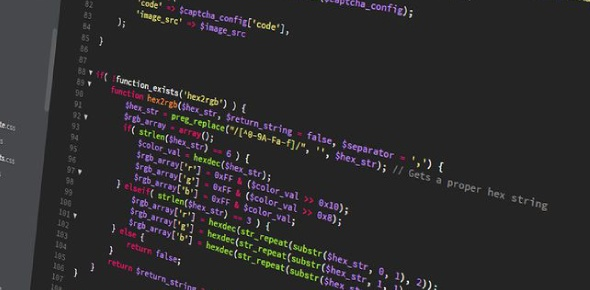# The Ultimate Python Programming MCQ Test

74 Questions | Total Attempts: 1253Settings• 1.
In Python, strings are…
• A.

Str objects

• B.

Char arrays

• C.

Changeable

• 2.
In Python, strings are...
• A.

Changeable

• B.

Char arrays

• C.

Immutable

• 3.
The minsplit parameter to split() specifies the minimum number of splits to make to the input string.
• A.

False

• B.

True

• 4.
Which of the following would separate a string input_string on the first 2 occurrences of the letter "e"?
• A.

'e'.split(input_string, 2)

• B.

Input_string.split('e', maxsplit=2)

• C.

'e'.split(input_string, maxsplit= 2)

• 5.
Which of the following would separate a string input_string on the first 2 occurrences of the letter "e"?
• A.

Input_string.split('e', 2)

• B.

'e'.split(input_string, maxsplit=2)

• C.

'e'.split(input_string, 2)

• 6.
Python strings have a property called “immutability”. What does this mean?
• A.

You can update a string in Python with concatenation

• B.

Strings in Python can be represented as arrays of char

• C.

Strings can’t be divided by numbers

• D.

Strings in Python can’t be changed

• 7.
If you want to transform a list of strings input_list into a single string with a comma between each item, which of the following would you give as the input to join()?
• A.

','

• B.

Input_list

• C.

Str

• D.

String

• 8.
Which of the following mathematical operators can be used to concatenate strings:
• A.

+

• B.

-

• C.

/

• 9.
Which of the following mathematical operators can be used to concatenate strings:
• A.

/

• B.

*

• C.

-

• D.

Option 4

• 10.
Assume x and y are assigned as follows: x=5, y=-5. What is the effect of this statement: x, y = (y, x)[::-1]
• A.

Both x and y are 5

• B.

The values of x and y are swaped

• C.

The values of x and y are unchanged

• D.

Both x and y are -5

• 11.
In regards to separated value files such as .csv and .tsv, what is the delimiter?
• A.

Any character such as the comma (,) or tab (\t) that is used to separate the row data

• B.

Delimiters are not used in separated value files

• C.

Anywhere the comma (,) character is used in the file

• D.

Any character such as the comma (,) or tab (\t) that is used to separate the column data

• 12.
In separated value files such as .csv and .tsv what does the first row in the file typically contain?
• A.

The author of the table data

• B.

The column names of the data

• C.

The source of the data

• D.

• 13.
Consider this assignment statement: a,b,c = (1,2,3,4,5,6,7,8,9)[1::3]. Following execution of this statement, what is the value of b:
• A.

2

• B.

4

• C.

5

• D.

6

• 14.
Which of the following is the correct way to open the CSV file hrdata.csv for reading using the pandas package? Assume that the pandas package has already been imported.
• A.

• B.

Pandas.open('hrdata.csv', 'r')

• C.

• D.

Pandas.open_csv('hrdata.csv', 'r')

• 15.
. What is a correct syntax to output “Hello World” in Python?
• A.

P("Hello, World")

• B.

Echo "Hello, World"

• C.

Echo ("Hello, World")

• D.

Print("Hello, World")

• 16.
How do you insert COMMENTS in Python code?
• A.

<--this is a comment-->

• B.

/*this is a comment*/

• C.

#this is a comment

• D.

//this is a comment//

• 17.
Which collection is ordered, changeable, and allows duplicate members?
• A.

TUPLE

• B.

SET

• C.

LIST

• D.

DICTIONARY

• 18.
1. Which of the following are true about python lists?
• A.

These represent the same list: ['a', 'b', 'c'] ['c', 'a', 'b']

• B.

All elements in a list must be of the same type

• C.

A given object may appear in a list more than once

• D.

There is no conceptual limit to the size of a list

• 19.
Which collection does not allow duplicate members?
• A.

TUPLE

• B.

SET

• C.

LIST

• 20.
1. Assume the following list definition:
>>> a = ['foo', 'bar', 'baz', 'qux', 'quux', 'corge'] Several short REPL sessions are shown below. Which display correct output?
• A.

· >>> print(a[4::-2]) ['quux', 'baz', 'foo']

• B.

· >>> a[:] is a True

• C.

· >>> print(a[-5:-3]) ['bar', 'baz']

• D.

· >>> max(a[2:4] + ['grault']) 'qux'

• E.

· >>> print(a[-6]) Traceback (most recent call last):   File "<stdin>", line 1, in <module> IndexError: list index out of range

• 21.
A collection which is unordered, changeable and does not allow duplicates.
• A.

TUPLE

• B.

SET

• C.

LIST

• D.

DICTIONARY

• 22.
A collection which is ordered and unchangeable.
• A.

TUPLE

• B.

SET

• C.

LIST

• D.

DICTIONARY

• 23.
How do you start writing an if statement in Python?
• A.

If x > y:

• B.

If x > y then:

• C.

If (x > y)

• 24.
How do you start writing a while loop in Python?
• A.

While x > y:

• B.

While (x > y)

• C.

X > y while {

• D.

While x>y {

• 25.
Select the ones you like List a is defined as follows: a = [1, 2, 3, 4, 5] Select all of the following statements that remove the middle element 3 from a so that it equals [1, 2, 4, 5]:
• A.

A=[ ]

• B.

A[2:3]=[ ]

• C.

A.remove(3)

• D.

A[2:2]=[ ]

• E.

Del a

Related TopicsBack to top